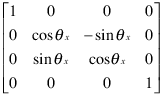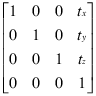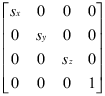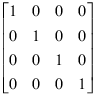## hgtransform 支持的变换

### 变换对象

#### 创建变换矩阵

`makehgtform` 函数简化了执行旋转、转换和缩放来构造矩阵的过程。有关创建使用 `makehgtform` 创建变换矩阵的详细信息，请参阅 嵌套变换，执行复杂移动

### 旋转### 转换### 缩放### 默认变换### 不允许的变换：透视

`$\left[\begin{array}{cccc}1& 0& 0& 0\\ 0& 1& 0& 0\\ 0& 0& 1& 0\\ 0& 0& {p}_{x}& 0\end{array}\right]$`

### 不允许的变换：剪切

`$\left[\begin{array}{cccc}1& {s}_{x}& 0& 0\\ 0& 1& 0& 0\\ 0& 0& 1& 0\\ 0& 0& 0& 1\end{array}\right]$`

### 将变换合并到一个矩阵

```C = R*T*S % operations are performed from right to left ```

`S` 是缩放矩阵，`T` 是转换矩阵，`R` 是旋转矩阵，`C` 是三种操作的复合矩阵。然后将变换对象的 `Matrix` 属性设置为 `C`

```hg = hgtransform('Matrix',C); ```

#### 将变换乘以单位矩阵

```hg.Matrix = C; hg.Matrix = eye(4);```

```I = eye(4); C = I*R*T*S; hg.Matrix = C;```

### 撤消变换操作

```hg = hgtransform('Matrix',C); ... hg.Matrix = eye(4);```